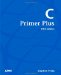# Summary

This chapter extended your knowledge about one-dimensional arrays from Chapter 10 to include arrays with several dimensions. In particular, the use of two- and three-dimensional arrays were discussed, along with their close connection to nested loops. The chapter further looked at selected useful built-in array properties, methods, and important algorithms for sorting and searching an array.

The essential points discussed in this chapter are reviewed in this section.

It is difficult to visualize more than three dimensions, but we don't need this ability, because the number of indexes required to reference an array element is equal to the number of dimensions of the array.

The values in a two-dimensional array can be thought of as a table with rows and columns. The first element in a two-dimensional array is at [0,0]. Conventionally, the elements of two-dimensional arrays are accessed as <Array_identifier>[<row>, <column>].

Array elements belonging to arrays of many dimensions can be used in expressions, just like other variables.

Use n nested loops to access all the elements of an n-dimensional array.

You can think of a two-dimensional array as an array of arrays. A rectangular array consists of a one-dimensional array with elements containing one-dimensional arrays of the same length. An array of two or more dimensions where every dimension consists of arrays of identical length is also a rectangular array.

A jagged array is an array with two or more dimensions where at least one of the dimensions consists of arrays that are not all of the same length.

A two-dimensional array is useful for representing the board (like the map in the Find the Island Game) of a board game.

The foreach construct makes it very simple to access a rectangular array of any dimension, it can be done with a non-nested foreach construct. However, this construct does not let you exert the same detailed control as the traditional loop statements (such as the for loop).

The foreach construct must be nested when used for accessing jagged arrays.

The array contains many useful built-in methods exerting functionality, such as sorting and searching.

Sorting and searching algorithms are used widely in the programming community.

The bubble sort algorithm is simple but slow and, therefore, is only useful to sort sets with a limited number of elements. The name bubble sort originates from the fact that smaller values gradually drift to the top (beginning) of an array during this search technique's execution.

The array's built-in sorting method is significantly faster than the bubble sort algorithm, especially when larger numbers of items are sorted.

The sequential search algorithm is simple and can be used on any set of items, but it is slow.

The binary search algorithm is very fast, but the set of items must be sorted prior to this search.

The array contains built-in search methods called IndexOf and LastIndexOf.C Primer Plus (5th Edition)
ISBN: 0672326965
EAN: 2147483647
Year: 2000
Pages: 286
Authors: Stephen Prata